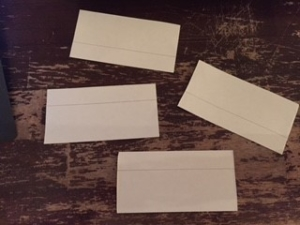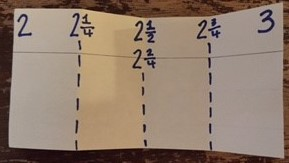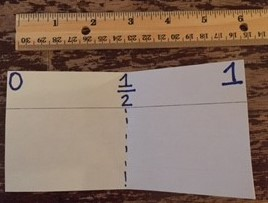Students in 3rd grade are expected to measure length to the nearest whole inch, half inch and quarter inch. (See the item specifications listed below).It is helpful to begin this learning by relating one whole inch to one whole on a number line. To do this, magnify one whole inch on a larger piece of paper to have students break the inch into fractional amounts by folding and relating to fractions on a number line. Follow the steps below:

1. Pass out 1 sentence strip to each student.

2. Have the students fold the sentence strip into fourths and cut.3. Then represent one of those pieces of paper as 1 whole inch “magnified.”4. Take one smaller piece of paper. Label the 0 and 1 on the paper, then fold down the middle. Pose the question, “What does this middle line represent?” Discuss how it is half of a whole inch so that mark represents ½ inch.5. Take another small piece of paper and fold down the middle, then fold in half again. Point to the first mark after 0 and pose the question, “What does this mark represent?” Have students explore and discuss that it represents 1 out of 4 spaces traveled on the paper so it’s ¼ of an inch. Continue this process with 2/4, ¾ and 1.6. Compare and contrast how we determined fractions of an inch to fractions on a number line in previous units. Pose a question such as, “How do the fractions of an inch compare to fractions of a whole?”7. Use the last piece of paper to explore a different range, such as 2-3 and fold to determine where 2 ½ would be located and other fractions within that range. Depending on how your students seem to be doing, may want to continue with other ranges to 12.8. Compare and contrast magnified inches to rulers – Pass out rulers to have students look for the fractions of a whole inch. Have them compare and contrast their paper magnified models to the actual ruler.For more information on developing understanding of fractions within a whole inch, visit the CPALMS Magnified Inch Lesson at http://www.cpalms.org/Public/PreviewResourceLesson/Preview/46871.